bendMitered

Create mitered bend shape on X-Y plane

Description

Use the bendMitered object to create a mitered bend shape on the X-Y plane.

Creation

Description

example

bend = bendMitered creates a mitered bend shape on the X-Y plane.

example

bend = bendMitered(Name=Value) sets Properties using one or more name-value arguments. For example, bendMitered(ReferencePoint=[1 1]) creates a mitered bend shape with the reference point at [1 1]. Properties not specified retain their default values.

Properties

expand all

Name of the mitered bend shape, specified as a character vector or a string scalar.

Example: bend = bendMitered(Name="bendmitered1")

Data Types: char

Reference point for the mitered bend shape in Cartesian coordinates, specified as a two-element vector.

Example: bend = bendMitered(ReferencePoint=[1 2])

Data Types: double

Length of the mitered bend shape in meters, specified as a two-element vector.

Example: bend = bendMitered(Length=[0.005 0.005])

Data Types: double

Width of the mitered bend shape in meters, specified as a two-element vector.

Example: bend = bendMitered(Width=[1 1])

Data Types: double

Length of the miter diagonal in meters, specified as a positive scalar.

Example: bend = bendMitered(MiterDiagonal=2)

Data Types: double

Object Functions

 add Boolean unite operation on two RF PCB shapes and Shape1 & Shape2 for RF PCB shapes area Calculate area of RF PCB shape in square meters intersect Boolean intersection operation on two RF PCB shapes mesh Change and view mesh properties of metal or dielectric in PCB component minus Shape1 - Shape2 for RF PCB shapes plus Shape1 + Shape2 for RF PCB shapes rotate Rotate RF PCB shape about defined axis rotateX Rotate RF PCB shape about x-axis rotateY Rotate RF PCB shape about y-axis and angle rotateZ Rotate RF PCB shape about z-axis subtract Boolean subtraction operation on two RF PCB shapes scale Change size of RF PCB shape by fixed amount show Display PCB component structure or PCB shape translate Move RF PCB shape to new location

Examples

collapse all

Create a mitered bend with default properties.

bend = bendMitered
bend =
bendMitered with properties:

Name: 'myMiteredbend'
ReferencePoint: [0 0]
Length: [0.0100 0.0100]
Width: [0.0050 0.0050]
MiterDiagonal: 0.0035

View the shape.

show(bend)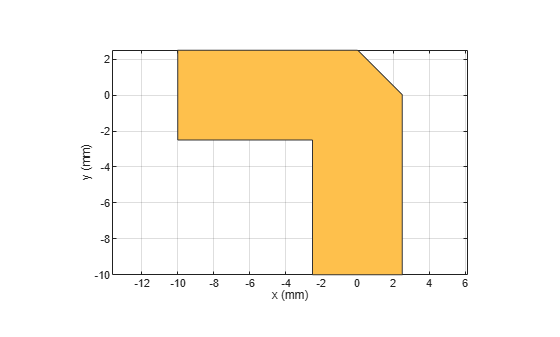Create a mitered bend shape of lengths of 10 m and 2 m, width of 2 m, and rotate it about the Z-axis by 60 degrees.

bend = bendMitered(Length=[10 2],Width=[2 2],MiterDiagonal=1);
bend = rotateZ(bend,60)
bend =
bendMitered with properties:

Name: 'myMiteredbend'
ReferencePoint: [0 0]
Length: [10 2]
Width: [2 2]
MiterDiagonal: 1

show(bend)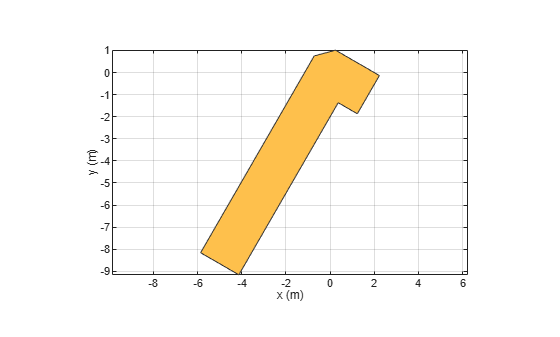Mesh the mitered bend shape at a maximum edge length of 1 m.

mesh(bend,MaxEdgeLength=1)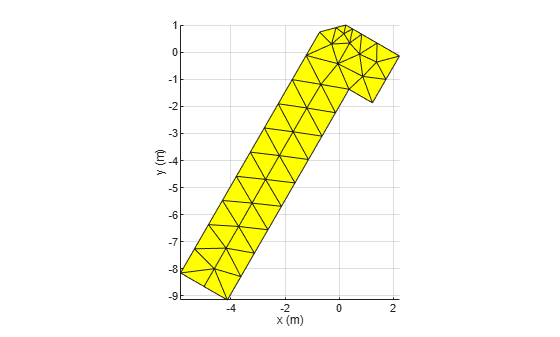Create mitered bend microstrip.

m = design(microstripLine,6e9,"Z0",75);
layer2d = bendMitered('Length',[m.Length/2 m.Length/2],...
"Width",[m.Width m.Width],'MiterDiagonal',sqrt(2)*m.Width);
robj = pcbComponent(layer2d);
robj.BoardThickness = m.Substrate.Thickness;
robj.Layers{2} = m.Substrate;
show(robj)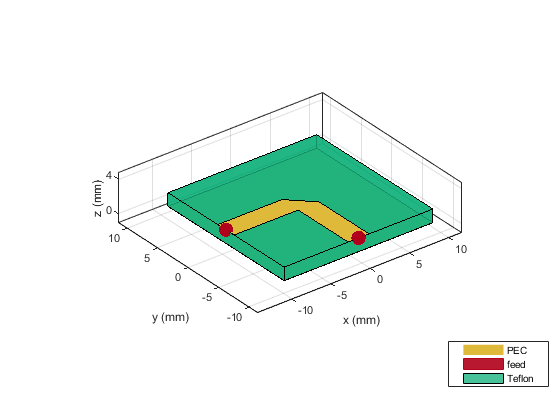Compute and plot s-parameters.

freq = (1:2:60)*100e6;
Sckt = sparameters(robj,freq,'Behavioral',true);
Sem = sparameters(robj,freq);
rfplot(Sckt,1,1,'db','-s')
hold on
rfplot(Sem,1,1,'db','-x')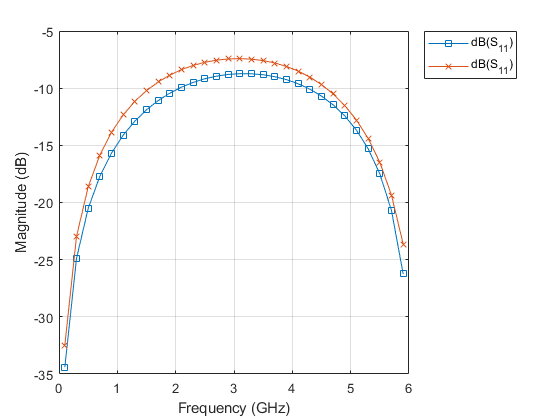Reference:

M. Kirschning, R. H. Jansen and N. H. L. Koster, "Measurement and Computer-Aided Modeling of Microstrip Discontinuities by an Improved Resonator Method," 1983 IEEE MTT-S International Microwave Symposium Digest, Boston, MA, USA, 1983, pp. 495-497, doi: 10.1109/MWSYM.1983.1130959.